# NCERT Solutions class 7 Maths Chapter-10 Exercise 10.2

NCERT Solutions Class-7 Maths chapter-10 line and angle Exercise-10.2 is prepared by academic team of pw all the questions of NCERT text book are solved step by step with proper and detail solutions explaining each and every questions . For More and additional questions of CBSE class 7 maths you can go to class 7 maths sections. NCERT class 7 Maths Solutions is the best way to enhanced your mathematics skill. And pw practice worksheet & question bank will help you a lot .

## NCERT Solutions class 7 Maths Chapter-10 line and angle

### Solutions of Chapter line and angle Exercise-10.2

Question 1:
Construct XYZ in which XY = 4.5 cm, YZ = 5 cm and ZX = 6 cm.
The rough figure of this triangle is as follows.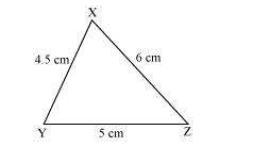The required triangle is constructed as follows.
(i) Draw a line segment YZ of length 5 cm.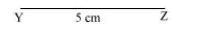(ii) Point X is at a distance of 4.5 cm from point Y. Therefore, taking point Y as centre, draw an arc of 4.5 cm radius.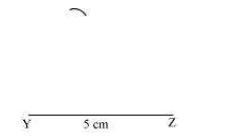(iii) Point X is at a distance of 6 cm from point Z. Therefore, taking point Z as centre, draw an arc of 6 cm radius. Mark the point of intersection of the arcs as X. Join XY and XZ.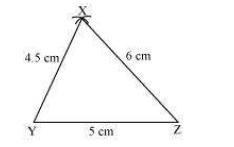XYZ is the required triangle.

Question 2:
Construct an equilateral triangle of side 5.5 cm.
An equilateral triangle of side 5.5 cm has to be constructed. We know that all sides of an equilateral triangle are of equal length. Therefore, a triangle ABC has to be constructed with AB = BC = CA = 5.5 cm. The steps of construction are as follows.
(i) Draw a line segment BC of length 5.5 cm.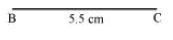(ii) Taking point B as centre, draw an arc of 5.5 cm radius.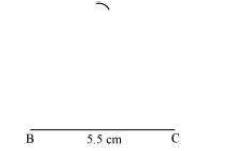(iii) Taking point C as centre, draw an arc of 5.5 cm radius to meet the previous arc at point A.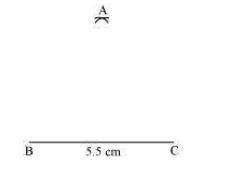(iv) Join A to B and C.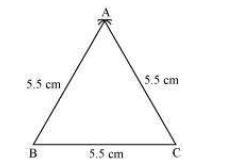ABC is the required equilateral triangle.

Question 3:
Draw PQR with PQ = 4 cm, QR = 3.5 cm and PR = 4 cm. What type of triangle is this?
The steps of construction are as follows.
(i) Draw a line segment QR of length 3.5 cm.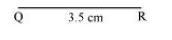(ii) Taking point Q as centre, draw an arc of 4 cm radius.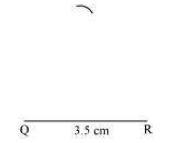(iii) Taking point R as centre, draw an arc of 4 cm radius to intersect the previous arc at point P.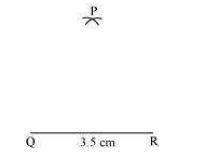(iv) Join P to Q and R.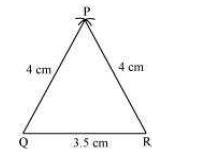PQR is the required triangle. As the two sides of this triangle are of the same length (PQ = PR), therefore, ?PQR is an isosceles triangle.

Question 4:
Construct ABC such that AB = 2.5 cm, BC = 6 cm and AC = 6.5 cm. Measure ∠B.
The steps of construction are as follows.
(i) Draw a line segment BC of length 6 cm.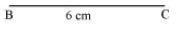(ii) Taking point C as centre, draw an arc of 6.5 cm radius.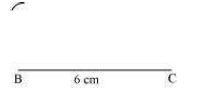(iii) Taking point B as centre, draw an arc of radius 2.5 cm to meet the previous arc at point A.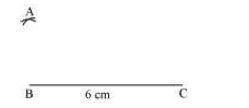(iv) Join A to B and C.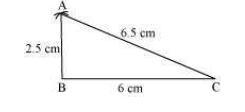ABC is the required triangle. ∠B can be measured with the help of protractor. It comes to 90º.

NCERT CLASS 7 MATHEMATICS SOLUTIONS